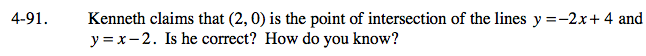### Home > CAAC > Chapter 4 > Lesson 4.2.3 > Problem4-91

4-91.Check his work by substituting (2, 0) into each equation.

y = −2x + 4
0 = −2(2) + 4

True or false?

Check the other equation.

(2, 0) is a solution to both equations, so it is where the two lines intersect.